Tuesday, September 19, 2006

PowARRRRRRR law?

International Talk Like A Pirate Day was everything I could have dreamed of and more. On a whim, I started comparing the popularity of different spellings of the canonical pirate interjection "ARRRRR". I did this systematically, doing Google searches on the letter A followed by any number of R's.

Obviously, the word "a" (the trivial case, which no one would ever use as a pirate interjection) was by far the most popular, with 19 billion hits. "Ar" (still not a common pirate interjection, but the standard abbreviation for arrrrrrrrgon, Arrrrrrrkansas, and Arrrrrrrrgentina) was also very popular, with 622 million. "Arr" is also an abbreviation for various things, and got 16 million hits. With "arrr", we enter pirate country, which continues with "arrrr", "arrrrr", and so forth.

After doing a number of these searches, it became clear that there was an monotonically decreasing, possibly exponential relationship between the number of R's and the number of Google hits. Here is the data plotted on logarithmic scale. Click on it for a larger version.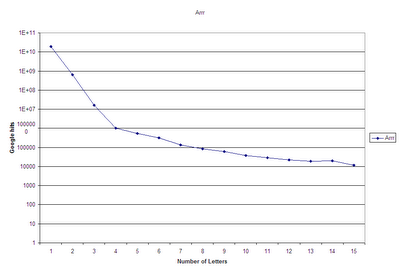So, as the number of hits steadily decreased, I figured I'd keep doing these Google searches until I reached a spelling that got no hits. I clearly underestimated the number and diversity of pirate imitators on the Internet. There were substantial numbers of hits for "arrrrrrrrrrrrrrrrrrrrrrrrrrrrrrrr" (32 R's) ("Did you mean: grrrrrrrrrrrrrrrrrrrrrrrrrrrrrrrr") "arrrrrrrrrrrrrrrrrrrrrrrrrrrrrrrrrrrrrrrrrrrrrrrrrrrrrrr" (55 R's) ("Did you mean: rrrrrrrrrrrrrrrrrrrrrrrrrrrrrrrrrrrrrrrrrrrrrrrrrrrrrrr"), and it just kept on going and going. After googlewhacks (1 solitary hit) at 101 and 104 R's, I finally reached the elusive goal at 105 R's. At that point I compiled the data: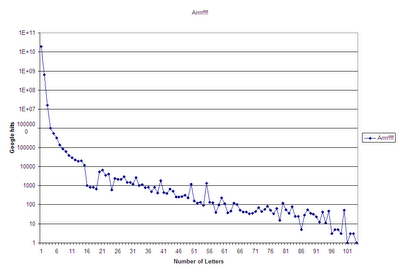Note that it looks exponential-ish, even though it's already graphed on a logarithmic scale. If it were actually an inverse exponential relationship, then this graph should be a straight line. We briefly considered the possibility that it could be a double exponential (e^e^-x), though I've never heard of any physical phenomenon with that type of relationship.

Next, I decided to plot it on a log-log graph, to see if it was a power-law relationship, as are so many other phenomena. (Does anyone know how to do this in Excel, other than by taking the log of each column directly? So that the axes will be labeled with the actual data points rather than with the logs of the data points?)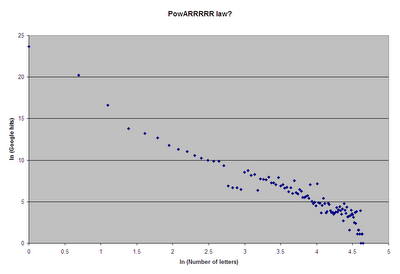Sure enough, it looks like something approximating a straight line! So the relationship between the number of Google hits (G) and the number of R's (R) can be described by a power law. Doing the regression in Excel, the best-fit curve is G = 657089947.6 * R^-3.886866982, with a correlation coefficient of 0.962. In other words, doubling the number of R's results in dividing the number of Google hits by about 15. Tripling the number of R's results in dividing the number of Google hits by about 72.

If anyone would like to do further analysis, I'm happy to send you the raw data - just email mahrabu at gmail. One unexplained phenomenon is the dearth of Google hits for 16 to 19 R's, only to return to the regular pattern with 20 and above. This provides new and exciting directions for further research in the field. Ahoy!

UPDATE: We've been linked from Language Log! Our study on "AR+" resembles a similar study on "AW+".

UPDATE 2: Wow, some of the prior research is incredible! Hat tip: Three-Toed Sloth

UPDATE 3: Thanks to a correspondent at the University of Minnesota for answering the Excel question. Here is a better-labeled graph: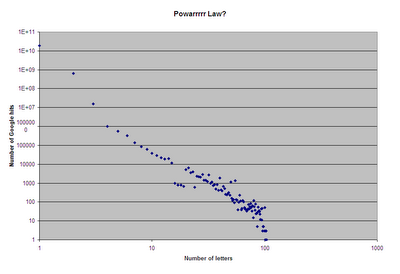1.What you really need now is a spectral analysis. Excel is a wimpy tool - I'd either examine it in MATLAB using their Fast Fourier Transform or code it directly in C or something like that. -DT

2.any difference between odd and even number of 'r's?

3.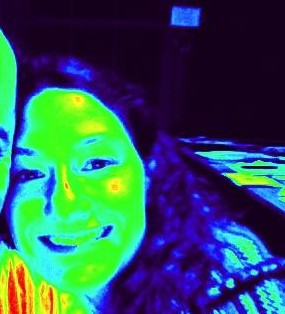fascinating! I looked into how multiple "A"s in the pirate word faired. The results were hard for me to interpret. For 2 and 3 "A"s the trend is to decrease as number of Rs increases but there is a wierd dip-- like the one you documented for nA=1, nR=16 through 19-- at nR=5 where hits at nR=5 is lower than hits at nR=10. The dip for nA=4 seems to happen at nR=3...

4.I agree that Excel isn't the way to go. I suggest using GNU R, or should I say 'GNU RRRRRRRRRRRR'. You'll find some R-related links here:

http://www.zapata.org/stuart/r/

5.You been MetaFiltered! Congrats!

6.I find a comparable-looking graph for "grrl", "grrrl", etc. No zeroes until R=50, and a weird spike at R=22. The power law in this case is G = 79717524.5*R^-4.4593, with correlation coefficient 0.9322.